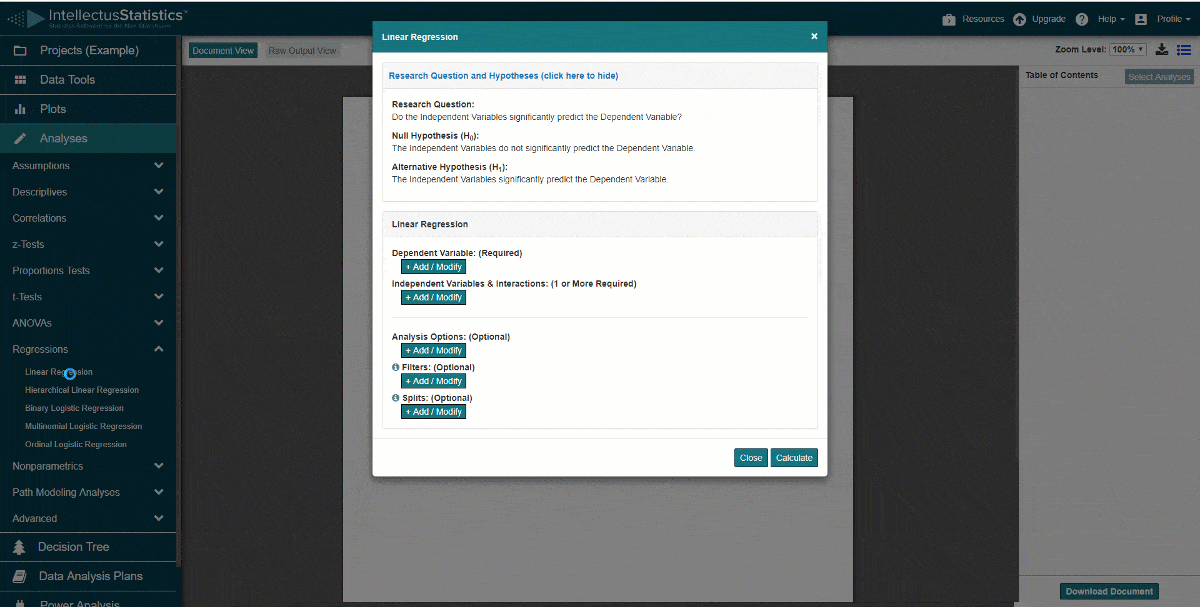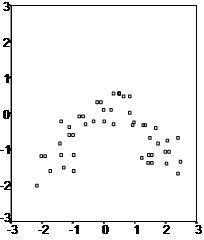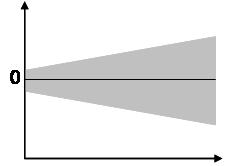# Assumptions of Linear Regression

Linear regression is an analysis that assesses whether one or more predictor variables explain the dependent (criterion) variable.  The regression has five key assumptions:

• Linear relationship
• Multivariate normality
• No or little multicollinearity
• No auto-correlation
• Homoscedasticity

A note about sample size.  In Linear regression the sample size rule of thumb is that the regression analysis requires at least 20 cases per independent variable in the analysis.

In the software below, its really easy to conduct a regression and most of the assumptions are preloaded and interpreted for you.# Quantitative Results in One-HourScreen share with a statistician as we walk you through conducting and understanding your interpreted analysis. Have your results draft complete in one hour with guaranteed accuracy.

First, linear regression needs the relationship between the independent and dependent variables to be linear.  It is also important to check for outliers since linear regression is sensitive to outlier effects.  The linearity assumption can best be tested with scatter plots, the following two examples depict two cases, where no and little linearity is present.Secondly, the linear regression analysis requires all variables to be multivariate normal.  This assumption can best be checked with a histogram or a Q-Q-Plot.  Normality can be checked with a goodness of fit test, e.g., the Kolmogorov-Smirnov test.  When the data is not normally distributed a non-linear transformation (e.g., log-transformation) might fix this issue.Thirdly, linear regression assumes that there is little or no multicollinearity in the data.  Multicollinearity occurs when the independent variables are too highly correlated with each other.

Multicollinearity may be tested with three central criteria:

1) Correlation matrix – when computing the matrix of Pearson’s Bivariate Correlation among all independent variables the correlation coefficients need to be smaller than 1.

2) Tolerance – the tolerance measures the influence of one independent variable on all other independent variables; the tolerance is calculated with an initial linear regression analysis.  Tolerance is defined as T = 1 – R² for these first step regression analysis.  With T < 0.1 there might be multicollinearity in the data and with T < 0.01 there certainly is.

3) Variance Inflation Factor (VIF) – the variance inflation factor of the linear regression is defined as VIF = 1/T. With VIF > 5 there is an indication that multicollinearity may be present; with VIF > 10 there is certainly multicollinearity among the variables.

If multicollinearity is found in the data, centering the data (that is deducting the mean of the variable from each score) might help to solve the problem.  However, the simplest way to address the problem is to remove independent variables with high VIF values.

Fourth, linear regression analysis requires that there is little or no autocorrelation in the data.  Autocorrelation occurs when the residuals are not independent from each other.  For instance, this typically occurs in stock prices, where the price is not independent from the previous price.

4) Condition Index – the condition index is calculated using a factor analysis on the independent variables.  Values of 10-30 indicate a mediocre multicollinearity in the linear regression variables, values > 30 indicate strong multicollinearity.

If multicollinearity is found in the data centering the data, that is deducting the mean score might help to solve the problem.  Other alternatives to tackle the problems is conducting a factor analysis and rotating the factors to insure independence of the factors in the linear regression analysis.

Fourthly, linear regression analysis requires that there is little or no autocorrelation in the data.  Autocorrelation occurs when the residuals are not independent from each other.  In other words when the value of y(x+1) is not independent from the value of y(x).While a scatterplot allows you to check for autocorrelations, you can test the linear regression model for autocorrelation with the Durbin-Watson test.  Durbin-Watson’s d tests the null hypothesis that the residuals are not linearly auto-correlated.  While d can assume values between 0 and 4, values around 2 indicate no autocorrelation.  As a rule of thumb values of 1.5 < d < 2.5 show that there is no auto-correlation in the data. However, the Durbin-Watson test only analyses linear autocorrelation and only between direct neighbors, which are first order effects.

The last assumption of the linear regression analysis is homoscedasticity.  The scatter plot is good way to check whether the data are homoscedastic (meaning the residuals are equal across the regression line).  The following scatter plots show examples of data that are not homoscedastic (i.e., heteroscedastic):The Goldfeld-Quandt Test can also be used to test for heteroscedasticity.  The test splits the data into two groups and tests to see if the variances of the residuals are similar across the groups.  If homoscedasticity is present, a non-linear correction might fix the problem.

Related Pages:

Linear Regression-Video Tutorial

Conduct and Interpret a Linear Regression